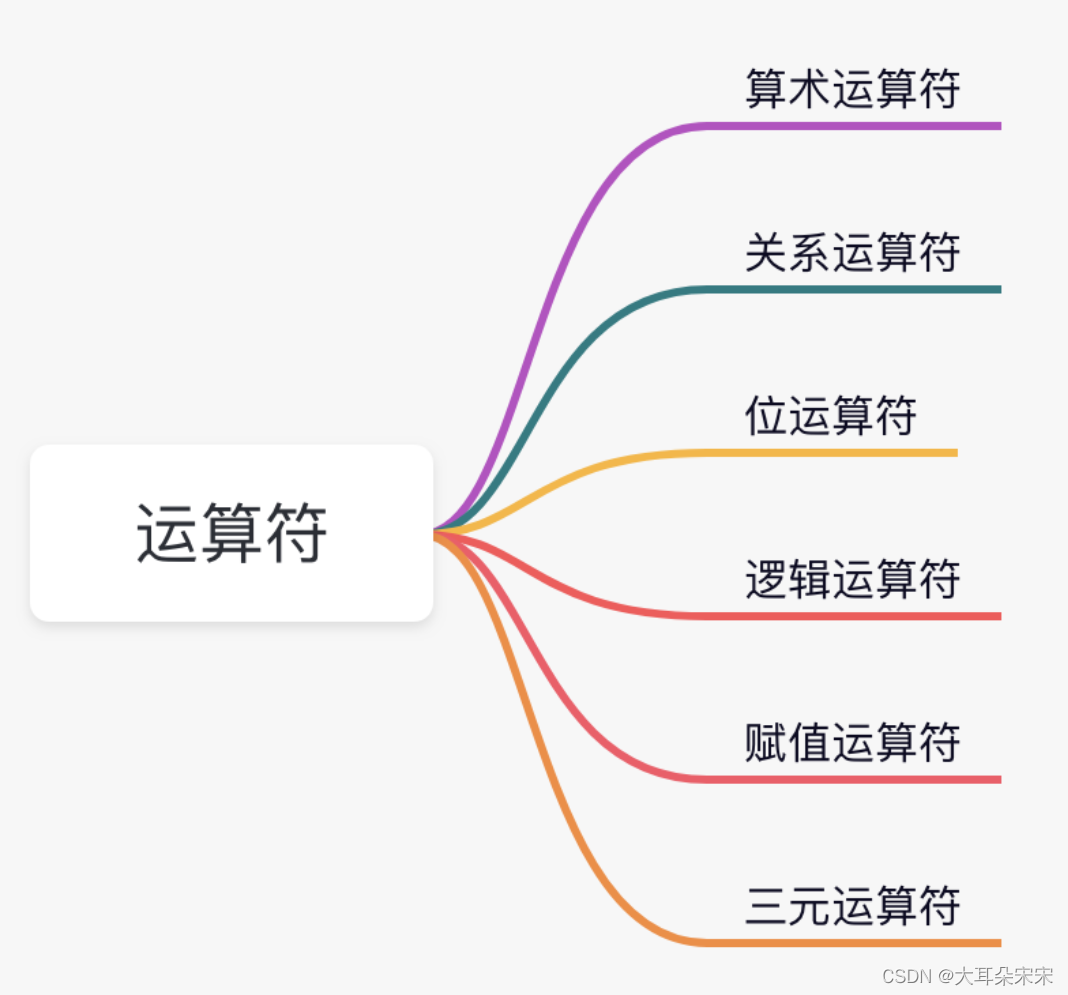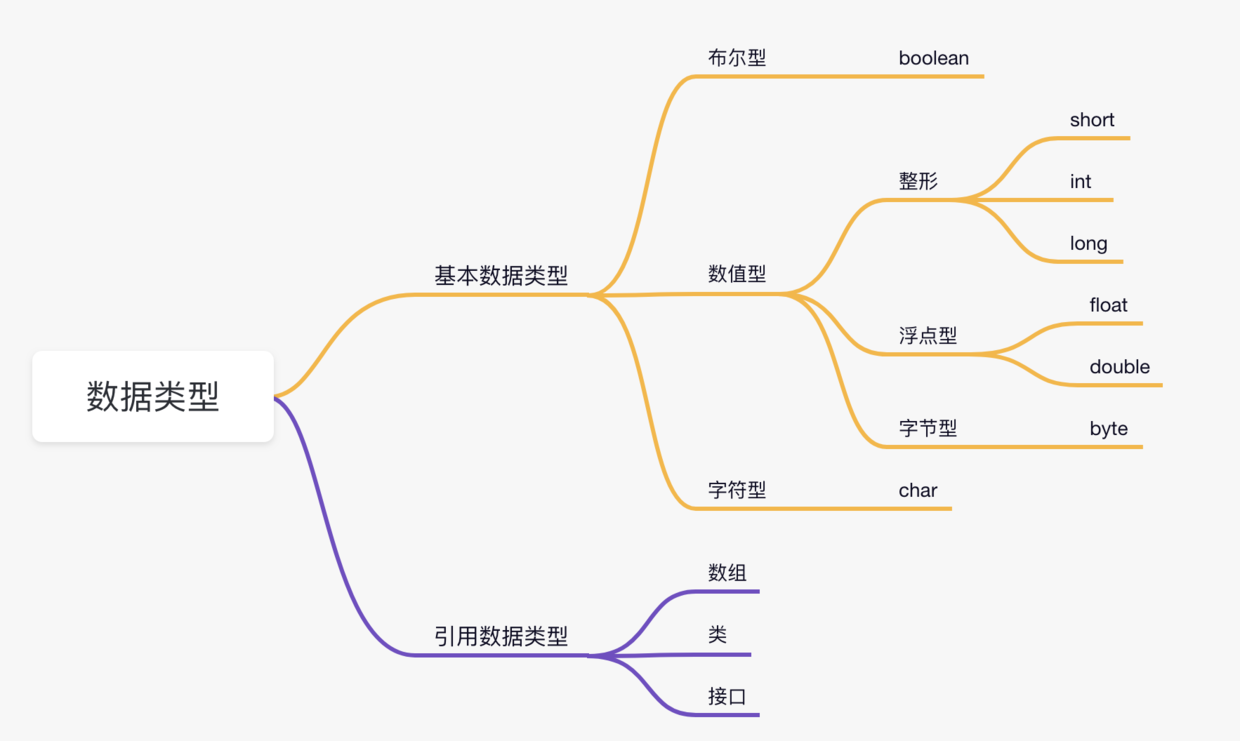+关注继续查看

• 算术运算符
• 关系运算符
• 位运算符
• 逻辑运算符
• 赋值运算符
• 其它运算符

# 算术运算符

+ 加法 - 相加运算符两侧的值 A + B 等于 30
- 减法 - 左操作数减去右操作数 A – B 等于 -10
* 乘法 - 相乘操作符两侧的值 A * B等于200
/ 除法 - 左操作数除以右操作数 B / A等于2

++ 自增: 操作数的值增加1 B++ 或 ++B 等于 21（区别详见下文）
-- 自减: 操作数的值减少1 B-- 或 --B 等于 19（区别详见下文）

### 实例

public class Test {

public static void main(String args[]) {
int a = 10;
int b = 20;
int c = 25;
int d = 25;
System.out.println("a + b = " + (a + b) );
System.out.println("a - b = " + (a - b) );
System.out.println("a * b = " + (a * b) );
System.out.println("b / a = " + (b / a) );
System.out.println("b % a = " + (b % a) );
System.out.println("c % a = " + (c % a) );
System.out.println("a++   = " +  (a++) );
System.out.println("a--   = " +  (a--) );
// 查看  d++ 与 ++d 的不同
System.out.println("d++   = " +  (d++) );
System.out.println("++d   = " +  (++d) );
}
}

## 自增自减运算符

1、自增（++）自减（--）运算符是一种特殊的算术运算符，在算术运算符中需要两个操作数来进行运算，而自增自减运算符是一个操作数。

public class selfAddMinus{
public static void main(String[] args){
int a = 3;//定义一个变量；
int b = ++a;//自增运算
int c = 3;
int d = --c;//自减运算
System.out.println("进行自增运算后的值等于"+b);
System.out.println("进行自减运算后的值等于"+d);
}
}

进行自增运算后的值等于4

• int b = ++a; 拆分运算过程为: a=a+1=4; b=a=4, 最后结果为b=4,a=4

• int d = --c; 拆分运算过程为: c=c-1=2; d=c=2, 最后结果为d=2,c=2

2、前缀自增自减法(++a,--a): 先进行自增或者自减运算，再进行表达式运算。

3、后缀自增自减法(a++,a--): 先进行表达式运算，再进行自增或者自减运算 实例：

public class selfAddMinus{
public static void main(String[] args){
int a = 5;//定义一个变量；
int b = 5;
int x = 2*++a;
int y = 2*b++;
System.out.println("自增运算符前缀运算后a="+a+",x="+x);
System.out.println("自增运算符后缀运算后b="+b+",y="+y);
}
}

自增运算符前缀运算后a=6，x=12

# 关系运算符

== 检查如果两个操作数的值是否相等，如果相等则条件为真。 （A == B）为假(非真)。
!= 检查如果两个操作数的值是否相等，如果值不相等则条件为真。 (A != B) 为真。

> = 检查左操作数的值是否大于或等于右操作数的值，如果是那么条件为真。 （A> = B）为假。
<= 检查左操作数的值是否小于或等于右操作数的值，如果是那么条件为真。 （A <= B）为真。

### 实例

public class Test {

public static void main(String args[]) {
int a = 10;
int b = 20;
System.out.println("a == b = " + (a == b) );
System.out.println("a != b = " + (a != b) );
System.out.println("a > b = " + (a > b) );
System.out.println("a < b = " + (a < b) );
System.out.println("b >= a = " + (b >= a) );
System.out.println("b <= a = " + (b <= a) );
}
}

a == b = false
a != b = true
a > b = false
a < b = true
b >= a = true
b <= a = false

# 位运算符

Java定义了位运算符，应用于整数类型(int)，长整型(long)，短整型(short)，字符型(char)，和字节型(byte)等类型。

A = 0011 1100
B = 0000 1101
-----------------
A&b = 0000 1100
A | B = 0011 1101
A ^ B = 0011 0001
~A= 1100 0011

| 如果相对应位都是0，则结果为0，否则为1 （A | B）得到61，即 0011 1101
^ 如果相对应位值相同，则结果为0，否则为1 （A ^ B）得到49，即 0011 0001

<<  按位左移运算符。左操作数按位左移右操作数指定的位数。 A << 2得到240，即 1111 0000
>>  按位右移运算符。左操作数按位右移右操作数指定的位数。 A >> 2得到15即 1111
>>>  按位右移补零操作符。左操作数的值按右操作数指定的位数右移，移动得到的空位以零填充。 A>>>2得到15即0000 1111

### 实例

public class TestA {
public static void main(String args[]) {
int a = 60; /* 60 = 0011 1100 */
int b = 13; /* 13 = 0000 1101 */
int c = 0;
c = a & b;       /* 12 = 0000 1100 */
System.out.println("a & b = " + c );

c = a | b;       /* 61 = 0011 1101 */
System.out.println("a | b = " + c );

c = a ^ b;       /* 49 = 0011 0001 */
System.out.println("a ^ b = " + c );

c = ~a;          /*-61 = 1100 0011 */
System.out.println("~a = " + c );

c = a << 2;     /* 240 = 1111 0000 */
System.out.println("a << 2 = " + c );

c = a >> 2;     /* 15 = 1111 */
System.out.println("a >> 2  = " + c );

c = a >>> 2;     /* 15 = 0000 1111 */
System.out.println("a >>> 2 = " + c );
}
} 

a & b = 12
a | b = 61
a ^ b = 49
~a = -61
a << 2 = 240
a >> 15
a >>> 15

# 逻辑运算符

&& 称为逻辑与运算符。当且仅当两个操作数都为真，条件才为真。 （A && B）为假。
| | 称为逻辑或操作符。如果任何两个操作数任何一个为真，条件为真。 （A | | B）为真。

### 实例

public class TestA {
public static void main(String args[]) {
boolean a = true;
boolean b = false;
System.out.println("a && b = " + (a&&b));
System.out.println("a || b = " + (a||b) );
System.out.println("!(a && b) = " + !(a && b));
}
}

a && b = false
a || b = true
!(a && b) = true

## 短路逻辑运算符

public class LuoJi{
public static void main(String[] args){
int a = 5;//定义一个变量；
boolean b = (a<4)&&(a++<10);
System.out.println("使用短路逻辑运算符的结果为"+b);
System.out.println("a的结果为"+a);
}
}

使用短路逻辑运算符的结果为false
a的结果为5

# 赋值运算符

= 简单的赋值运算符，将右操作数的值赋给左侧操作数 C = A + B将把A + B得到的值赋给C
+ = 加和赋值操作符，它把左操作数和右操作数相加赋值给左操作数 C + = A等价于C = C + A
- = 减和赋值操作符，它把左操作数和右操作数相减赋值给左操作数 C - = A等价于C = C -
A
* = 乘和赋值操作符，它把左操作数和右操作数相乘赋值给左操作数 C * = A等价于C = C * A
/ = 除和赋值操作符，它把左操作数和右操作数相除赋值给左操作数 C / = A等价于C = C / A
（％）= 取模和赋值操作符，它把左操作数和右操作数取模后赋值给左操作数 C％= A等价于C = C％A
<< = 左移位赋值运算符 C << = 2等价于C = C << 2
>> = 右移位赋值运算符 C >> = 2等价于C = C >> 2
＆= 按位与赋值运算符 C＆= 2等价于C = C＆2
^ = 按位异或赋值操作符 C ^ = 2等价于C = C ^ 2
| = 按位或赋值操作符 C | = 2等价于C = C | 2

### 实例

public class TestA {
public static void main(String args[]) {
int a = 10;
int b = 20;
int c = 0;
c = a + b;
System.out.println("c = a + b = " + c );
c += a ;
System.out.println("c += a  = " + c );
c -= a ;
System.out.println("c -= a = " + c );
c *= a ;
System.out.println("c *= a = " + c );
a = 10;
c = 15;
c /= a ;
System.out.println("c /= a = " + c );
a = 10;
c = 15;
c %= a ;
System.out.println("c %= a  = " + c );
c <<= 2 ;
System.out.println("c <<= 2 = " + c );
c >>= 2 ;
System.out.println("c >>= 2 = " + c );
c >>= 2 ;
System.out.println("c >>= a = " + c );
c &= a ;
System.out.println("c &= 2  = " + c );
c ^= a ;
System.out.println("c ^= a   = " + c );
c |= a ;
System.out.println("c |= a   = " + c );
}
} 

c = a + b = 30
c += a  = 40
c -= a = 30
c *= a = 300
c /= a = 1
c %= a  = 5
c <<= 2 = 20
c >>= 2 = 5
c >>= 2 = 1
c &= a  = 0
c ^= a   = 10
c |= a   = 10

# 条件运算符（?:）

variable x = (expression) ? value if true : value if false

### 实例

public class Test {
public static void main(String args[]){
int a , b;
a = 10;
// 如果 a 等于 1 成立，则设置 b 为 20，否则为 30
b = (a == 1) ? 20 : 30;
System.out.println( "Value of b is : " +  b );

// 如果 a 等于 10 成立，则设置 b 为 20，否则为 30
b = (a == 10) ? 20 : 30;
System.out.println( "Value of b is : " + b );
}
}

Value of b is : 30
Value of b is : 20

# instanceof 运算符

instanceof运算符使用格式如下：

( Object reference variable ) instanceof  (class/interface type)

String name = "James";
boolean result = name instanceof String; // 由于 name 是 String 类型，所以返回真

class Vehicle {}

public class Car extends Vehicle {
public static void main(String args[]){
Vehicle a = new Car();
boolean result =  a instanceof Car;
System.out.println( result);
}
}

true

# Java运算符优先级Java修饰符和运算符，超详细整理，适合新手入门
Java修饰符和运算符，超详细整理，适合新手入门
14 0Java基础——运算符
Java提供的一组丰富的算术和逻辑运算符
17 0java运算符实例
java运算符实例
11 0java运算符
java运算符
14 0Java入门程序——Java运算符

42 0java运算符
java运算符
16 0【Java基础知识 4】Java数据类型之间的转换、运算符(2)
【Java基础知识 4】Java数据类型之间的转换、运算符(2)
20 0【Java基础知识 4】Java数据类型之间的转换、运算符（1）
【Java基础知识 4】Java数据类型之间的转换、运算符(1）
49 0【Java编程进阶】运算符与表达式

42 0

KARL-Dujinyang-奥特曼超人。作者目前任职于银狐、泡椒游戏公司，曾任职腾为CTO，CSDN技术专家，曾创办过创业go、造梦工厂、米奇云，万能孵化器等，领域涉及逆向安全专家以及全栈工程师，作者目前出书中。

Java面向对象开发23678106

Java高级开发31514156

Java Web开发系列课程 - Struts2框架入门49249

Java Web开发系列课程 - Spring框架入门2976925

Java Web开发-Web应用、Tomcat、HTTP请求与响应137017

Java Spring Boot 2.6.0开发实战-1024程序员节创造营公益课9295

Java工程师必读手册

Java应用提速（速度与激情）

Java单元测试实战